## Mining Frequent Closed Subgraphs in a Graph Database using the cgSpan algorithm (SPMF documentation)

This page explains how to run the cgSPAN algorithm using the SPMF open-source data mining library.

## What is this algorithm?

cgSpan is a fast algorithm for discovering frequent closed subgraphs either (1) in a graph database or (2) in a single large graph. The algorithm was proposed by Shaul et al. (2021).

There are several algorithms for discovering frequent subgraphs such as gSpan and TKG to name a few. The advantage of cgSpan over traditional subgraph mining algorithms is that it finds only the frequent closed subgraphs. The closed subgraphs are interesting because they provide a summary of all frequent subgraphs that is often much smaller than the set of all subgraphs, and it can be shown that no information is lost. Moreover, mining frequent closed subgraphs can be much faster than mining all frequent subgraphs. cgSpan is one of the most efficient algorithms for mining closed subgraphs, and is based on gSpan.

The implementation of cgSpan let you choose between two ways of counting the occurrences of subbgraphs. The first one is called is called "support" and is the traditional definition, used for mining subgraphs in a graph database. The second definition is the MNI support, which is adapted for mining subgraphs in a single large graph but can also be used for a graph database. Below, the two versions are described.

## A) Example 1: Finding Subgraphs in a Graph Database using the traditional definition of support

### How to run this example?

If you want to mine patterns using the traditional definition of support in a graph database:

• If you are using the graphical interface, (1) choose the "CGSPAN" algorithm, (2) select the input file "contextTKG.txt", (3) set the output file name (e.g. "output.txt") (4) set minsup to 90% and (5) click "Run algorithm".
• If you want to execute this example from the command line, then execute this command:
java -jar spmf.jar run CGSPAN contextTKG.txt output.txt 90%
in a folder containing spmf.jar and the example input file contextTKG.txt.
• If you are using the source code version of SPMF, launch the file "MainTestCGSPANSupport.java" in the package ca.pfv.SPMF.tests.

### What is the input of the algorithm?

The input is a set of labeled connected graphs and a threshold named minsup (a value between 0 and 100 %). Moreover, a few optional parameters can be set, which will be described further down this page.

To explain the input more clearly, some definitions are first introduced. A labeled graph is a set of vertices and edges, having some labels. Let’s me illustrate this idea with an example. Consider the following graph:This graph contains four vertices (depicted as yellow circles). These vertices have labels such as “10” and “11”.  These labels provide information about the vertices. For example, imagine that this graph is a  chemical molecule. The label 10 and 11 could represent the chemical elements of Hydrogen and Oxygen, respectively. Labels do not need to be unique. In other words, the same labels may be used to describe several vertices in the same graph. For example, if the above graph represents a chemical molecule, the labels “10” and “11” could be used for all vertices representing Oxygen and Hydrogen, respectively.

Now, besides vertices, a graph also contains edges. The edges are the lines between the vertices, here represented by thick black lines.  Edges also have some labels. In this example, four labels are used, which are 20, 21, 22 and 23.  These labels represents different types of relationships between vertices. Edge labels do not need to be unique. A graph is a connected graph if by following the edges, it is possible to go from any vertex to any other vertices.

The goal of frequent subgraph mining is to discover interesting subgraph(s) appearing in a set of graphs (a graph database). But how can we judge if a subgraph is interesting?  This depends on the application. The interestingness can be defined in various ways. Traditionally, a subgraph has been considered as interesting if it appears multiple times in a set of graphs. In other words, we want to discover subgraphs that are common to multiple graphs. This can be useful for example to find association between chemical elements common to several chemical molecules.

The algorithm takes a graph database as input. Then, a frequent subgraph mining algorithm will enumerate as output all frequent subgraphs. A frequent subgraph is a subgraph that appears in at least minsup percents of the graphs from the graph database.  For example, let’s consider the following graph database containing three graphs, which is provided in the file contextTKG.txt of the SPMF distribution.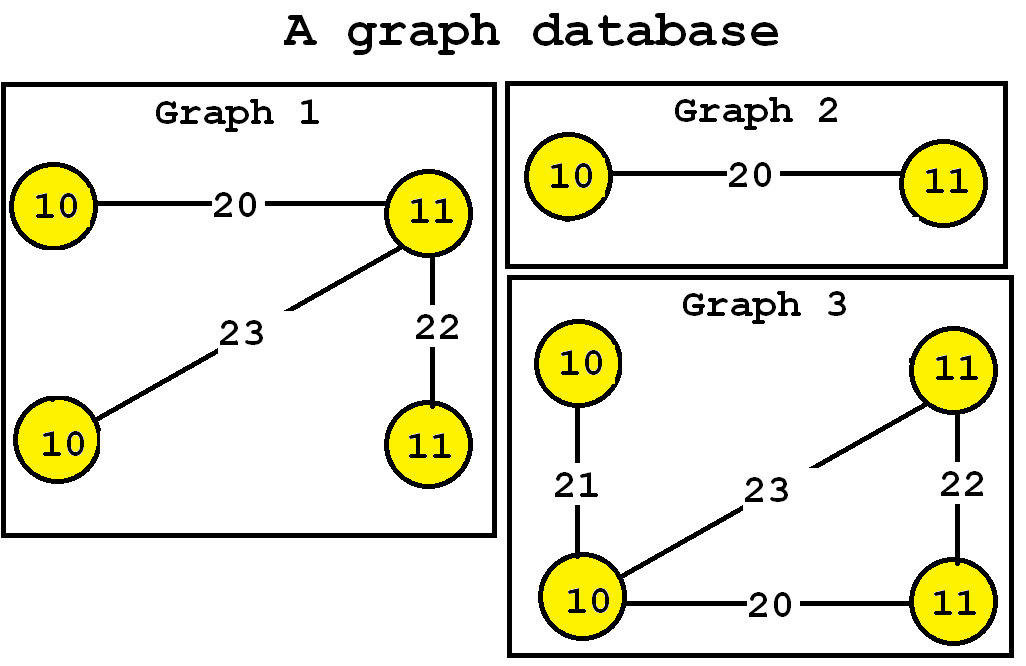This database contains three graphs, respectively called Graph 1, 2 and 3.

### What is the output of the algorithm?

The output of a traditional frequent subgraph mining algorithm is the set of all frequent subgraphs. A frequent subgraph is a subgraph that appears in at least minsup percent of the graphs of the input graph database, and their support values. In this example, consider that the minsup parameter is set to 90 % (which means that frequent subgraphs must appear in at least 3 graphs of the input database since 90 % * 3 = 3). If we apply a traditional subgraph mining algorithm like gSpan, then three frequent subgraphs would be found:Consider the third subgraph (“Frequent subgraph 3”).  This subgraph is frequent and is said to have a support (a frequency) of 3 since it appears in three of the input graphs. These occurrences are highlighted in red, below: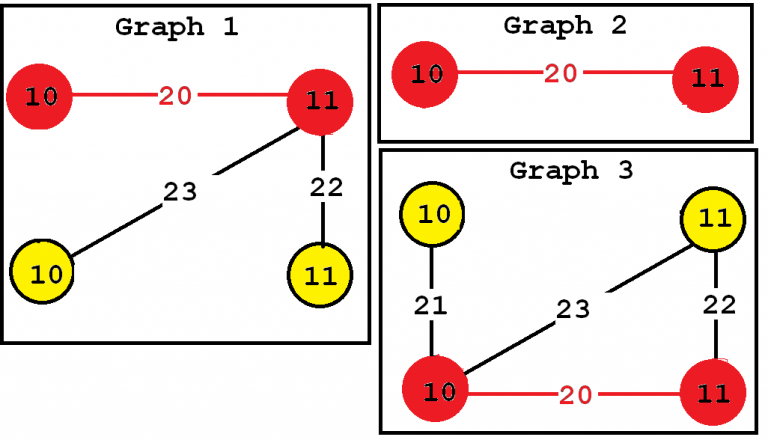In this example "Frequent subgraph 1" and "Frequent subgraph 2" also have a support of 3. Note that the support of a graph can be also expressed as a percentage. Since these subgraphs appear in three input graphs and the input database contains three graphs, the support of these subgraphs expressed as a percentage is 3 / 3 = 1 (which means 100 %).

But a problem with traditional frequent subgraph mining algorithms is that a very large number of subgraphs may be found, and some can be viewed as redundant.

To address this problems,  cgSpan only discovers the frequent closed subgraphs rather than all frequent subgraphs.

A frequent closed subgraph is a frequent subgraph that has no supergraph having the same support. It can be shown that by only discovering the closed subgraphs, a much smaller result set can be usually found compared to all frequent subgraphs and that no information is loss. The set of frequent closed subgraphs for this example is: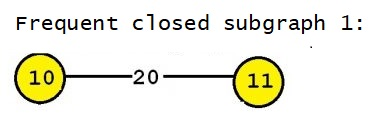Thus, cgSpan outputs a single frequent closed subgraph instead of the three frequent subgraphs.

The two key advantages of mining frequent closed subgraphs is that (1) it is often faster than mining all frequent subgraphs, and (2) no information is loss (all frequent subgraphs could be derived from the frequent closed subgraphs).

### Optional parameters

This implementation of cgSpan also has five optional parameters:

• maxNumberOfEdges : the maximum number of edges that frequent subgraphs should contain. This can be used to reduce the search space and find less frequent subgraphs.
• outputSingleFrequentVertices: if this parameter is set to true (by default), then frequent subgraphs containing a single vertex will also be output. Otherwise, not.
• outputDotFile: if this parameter is set to true (by default : false), then a DOT file will be also created as output. A DOT is a GraphViz file that can be used to visualize the frequent subgraphs using GraphViz
• outputGraphIds: if this parameter is set to true (by default: false), then the output file will indicate for each frequent subgraph, the list of input graphs where the subgraph appears.

The optional parameter can be specified in the graphical user interface of SPMF, but also when running SPMF from the command line. For example, to call cgSpan from the command line with minsup = 0.9, maxNumberOfEdges = 2, outputSingleFrequentVertices = true, outputDotFile = false, outputGraphIds = true, and outputDebugInformation = true, the command can be written as follows:

java -jar spmf.jar run CGSPAN contextTKG.txt output.txt 0.9 2 true false true true

### Input file format

The input file format is defined as follows. It is a text file which contains one or more graphs.  A graph is defined by a few lines of text that follow the following format:

• t # N    This is the first line of a graph. It indicates that this is the N-th graph in the file
• v M L     This line defines the M-th vertex of the current graph, which has a label L
• e P Q L   This line defines an edge, which connects the P-th vertex with the Q-th vertex. This edge has the label L

For the above example, the input file is defined as follows:

t # 0
v 0 10
v 1 11
v 2 10
v 3 11
e 0 1 20
e 1 2 23
e 1 3 22

t # 1
v 4 10
v 5 11
e 4 5 20

t # 2
v 6 10
v 7 10
v 8 11
v 9 11
e 6 7 21
e 7 8 23
e 7 9 20
e 8 9 22

### Output file format

The output file format is defined as follows. It is a text file, listing all the frequent subgraphs found in the input graph database. A frequent subgraph is defined by a few lines of text that follow the following format:

• t # N * Z    This is the first line of a subgraph. It indicates that this is the N-th subgraph in the file and that its support is Z.
• v M L     This line defines the M-th vertex of the current subgraph, which has a label L
• e P Q L   This line defines an edge, which connects the P-th vertex with the Q-th vertex. This edge has the label L
• x X1 X2 ... This line lists the identifiers of all the graphs X1, X2 ... that contains the current subgraph.
For example, for the above example, the output is:

t # 0 * 3
v 0 10
v 1 11
e 0 1 20
x 0 1 2

If the optional parameter outputDebugInformation is activated (set to true) and outputGraphIds is activated as well, then additional information is included in the output. In that case the format is:
• t # N * Z *T    This is the first line of a subgraph. It indicates that this is the N-th subgraph in the file and that its support is Z. Moreover, it is indicates that the graph has T projections in the database)
• v M L     This line defines the M-th vertex of the current subgraph, which has a label L
• e P Q L   This line defines an edge, which connects the P-th vertex with the Q-th vertex. This edge has the label L
• x A1xB1 A2xB2 ... This line contains "x" followed by a list of pairs of the form AxB where A is the ID of a graph containing the subgraph, and B is the number of projections of the subgraph in graph A.

For example, for the above example the output when outputDebugInformation = true  and outputGraphIds = true, is :

t # 0 * 3 * 3
v 0 10
v 1 11
e 0 1 20
x 0x1 1x1 2x1

And if outputDebugInformation = true  and outputGraphIds = false, the output is :

t # 0 * 3 * 3
v 0 10
v 1 11
e 0 1 20

## B) Example 2: Finding Subgraphs in a Graph Database using the MNI support

If you want to mine patterns using the MNI definition of support in a single graph or graph database

• If you are using the graphical interface, (1) choose the "CGSPAN_MNI" algorithm, (2) select the input file "contextTKG.txt", (3) set the output file name (e.g. "output.txt") (4) set minNMI to 3 and (5) click "Run algorithm".
• If you want to execute this example from the command line, then execute this command:
java -jar spmf.jar run contextTKG.txt output.txt 3
in a folder containing spmf.jar and the example input file contextTKG.txt.
• If you are using the source code version of SPMF, launch the file "MainTestCGSPANMNI.java" in the package ca.pfv.SPMF.tests.

### What is the input of the algorithm?

The input is a one or more labeled connected graphs and a threshold named minMNI (an integer no less than zero). Moreover, a few optional parameters can be set, which will be described further down this page.

In this example, we will show the application of this algorithm for a graph database as input. Then the f requent subgraph mining algorithm will enumerate as output all frequent subgraphs. A frequent subgraph is a subgraph that has at least some minimum number of occurrences (suport) in the input graphs.  For example, let’s consider the following graph database containing three graphs, which is provided in the file contextTKG.txt of the SPMF distribution.This database contains three graphs, respectively called Graph 1, 2 and 3.

This graph contains four vertices (depicted as yellow circles). These vertices have labels such as “10” and “11”.  These labels provide information about the vertices. For example, imagine that this graph is a chemical molecule. The label 10 and 11 could represent the chemical elements of Hydrogen and Oxygen, respectively. Labels do not need to be unique. In other words, the same labels may be used to describe several vertices in the same graph. For example, if the above graph represents a chemical molecule, the labels “10” and “11” could be used for all vertices representing Oxygen and Hydrogen, respectively.

Now, besides vertices, a graph also contains edges. The edges are the lines between the vertices, here represented by thick black lines.  Edges also have some labels. In this example, four labels are used, which are 20, 21, 22 and 23.  These labels represents different types of relationships between vertices. Edge labels do not need to be unique. A graph is a connected graph if by following the edges, it is possible to go from any vertex to any other vertices.

The goal of frequent subgraph mining in a single graph or a graph database is to discover interesting subgraph(s) that have many occurrences. But how can we judge if a subgraph is interesting?  This depends on the application. The interestingness can be defined in various ways. Traditionally, a subgraph has been considered as interesting if it appears multiple times. This can be multiple times in a single graph or in a set of graph (a graph database).

There are different ways to count the number of occurrences that a subgraph has. In the first example in this page, we have explained the traditional definition of support. In this example, we will now explain an alternative way of counting the occurrences called the MNI support. The advantage of the MNI support is that it can count multiple occurrences in a same graph (which may be overlapping). An explanation of the MNI support will be given further below.

The algorithm takes a graph database as input. Then, the cgSpan frequent subgraph mining algorithm will enumerate as output all frequent closed subgraphs. A frequent subgraph is a subgraph if its MNI support is no less than the threshold minMNI  deffined by the user. A frequent subgraph is closed if it is not included in another subgraph having the same support.

### What is the output of the algorithm?

The output is the set of all frequent closed subgraphs. A frequent subgraph is a subgraph that has a MNI support of at least minMNI in the input graph(s). In this example, consider that the minMNI parameter is set to 3 (which means that frequent subgraphs must have a MNI support of at least 2 in the input database). A frequent subgraph is closed if it is not included in another subgraph having the same support.

The output of cgSpan in this case is: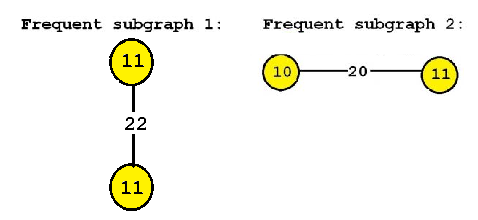These two subgraphs have a MNI support of 4 and 3 respectively. Their occurrences in the input graph database according to the MNI support are: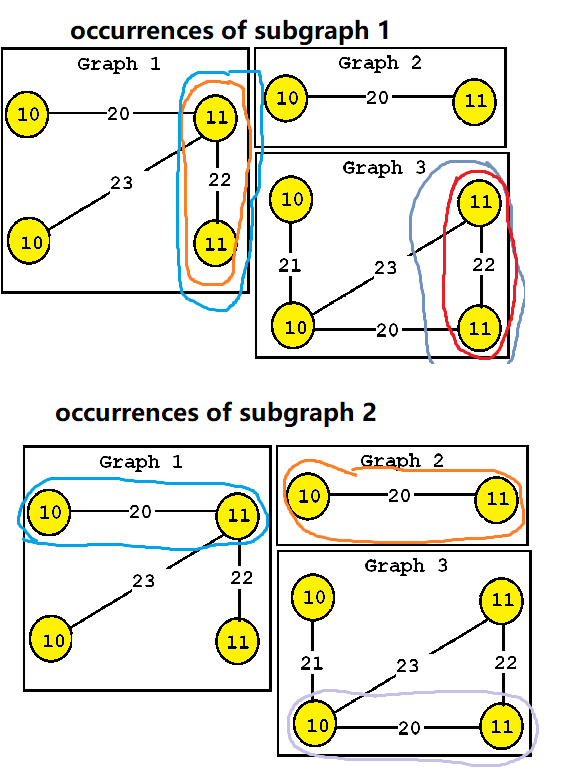### Output file format

The output file format is defined as follows. It is a text file, listing all the frequent subgraphs found in the input graph database. A frequent subgraph is defined by a few lines of text that follow the following format:

• t # N * Z    This is the first line of a subgraph. It indicates that this is the N-th subgraph in the file and that its support is Z.
• v M L     This line defines the M-th vertex of the current subgraph, which has a label L
• e P Q L   This line defines an edge, which connects the P-th vertex with the Q-th vertex. This edge has the label L
• x X1 X2 ... This line lists the identifiers of all the graphs X1, X2 ... that contains the current subgraph.
For example, for the above example, the output is:

t # 0 * 4
v 0 11
v 1 11
e 0 1 22
x 0 2

t # 1 * 3
v 0 10
v 1 11
e 0 1 20
x 0 1 2

If the optional parameter outputDebugInformation is activated (set to true) and outputGraphIds is activated as well, then additional information is included in the output. In that case the format is:

• t # N * Z *T    This is the first line of a subgraph. It indicates that this is the N-th subgraph in the file and that its support is Z. Moreover, it is indicates that the graph has T projections in the database)
• v M L     This line defines the M-th vertex of the current subgraph, which has a label L
• e P Q L   This line defines an edge, which connects the P-th vertex with the Q-th vertex. This edge has the label L
• x A1xB1 A2xB2 ... This line contains "x" followed by a list of pairs of the form AxB where A is the ID of a graph containing the subgraph, and B is the number of projections of the subgraph in graph A.

For example, for the above example the output when outputDebugInformation = true  and outputGraphIds = true, is :

t # 0 * 4 * 4
v 0 11
v 1 11
e 0 1 22
x 0x2 2x2

t # 1 * 3 * 3
v 0 10
v 1 11
e 0 1 20
x 0x1 1x1 2x1

And if outputDebugInformation = true  and outputGraphIds = false, the output is :

t # 0 * 4 * 4
v 0 11
v 1 11
e 0 1 22

t # 1 * 3 * 3
v 0 10
v 1 11
e 0 1 20

## C) Example 2: Finding Subgraphs in Single Graph using the MNI support

If you want to mine patterns using the MNI definition of support in a single graph or graph database

• If you are using the graphical interface, (1) choose the "CGSPAN_MNI" algorithm, (2) select the input file "contextSingleGraph.txt", (3) set the output file name (e.g. "output.txt") (4) set minNMI to 2, maximum number of edges to 4 and (5) click "Run algorithm".
• If you want to execute this example from the command line, then execute this command:
java -jar spmf.jar run CGSPANcontextSingleGraph.txt output.txt 2 4
in a folder containing spmf.jar and the example input file contextSingleGraph.txt.
• If you are using the source code version of SPMF, launch the file "MainTestCGSPANMNI_singleGraph.java" in the package ca.pfv.SPMF.tests.

### What is the input of the algorithm?

The input is a one or more labeled connected graphs and a threshold named minMNI (an integer no less than zero). Moreover, a few optional parameters can be set, which will be described further down this page.

To explain this example, we will use a single graph, which is provided in the file "contextSingleGraph.txt" :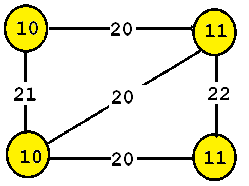This graph contains four vertices (depicted as yellow circles). These vertices have labels such as “10” and “11”.  These labels provide information about the vertices. For example, imagine that this graph is a chemical molecule. The label 10 and 11 could represent the chemical elements of Hydrogen and Oxygen, respectively. Labels do not need to be unique. In other words, the same labels may be used to describe several vertices in the same graph. For example, if the above graph represents a chemical molecule, the labels “10” and “11” could be used for all vertices representing Oxygen and Hydrogen, respectively.

Now, besides vertices, a graph also contains edges. The edges are the lines between the vertices, here represented by thick black lines.  Edges also have some labels. In this example, four labels are used, which are 20, 21, 22 and 23.  These labels represents different types of relationships between vertices. Edge labels do not need to be unique. A graph is a connected graph if by following the edges, it is possible to go from any vertex to any other vertices.

The goal of frequent subgraph mining in a single graph or a graph database is to discover interesting subgraph(s) that have many occurrences. But how can we judge if a subgraph is interesting?  This depends on the application. The interestingness can be defined in various ways. Traditionally, a subgraph has been considered as interesting if it appears multiple times. This can be multiple times in a single graph or in a set of graph (a graph database).

There are different ways to count the number of occurrences that a subgraph has. In the first example in this page, we have explained the traditional definition of support. In this example, we will now explain an alternative way of counting the occurrences called the MNI support. The advantage of the MNI support is that it can count multiple occurrences in a same graph (which may be overlapping). Because of this, the MNI support is suitable to find frequent subgraphs in a single graph unlike the traditional support measure which is only suitable to analyze graph databases. An explanation of the MNI support will be given further below.

The algorithm takes a graph database as input. Then, the cgSpan frequent subgraph mining algorithm will enumerate as output all frequent subgraphs. A frequent subgraph is a subgraph if its MNI support is no less than the threshold minMNI  deffined by the user.

### What is the output of the algorithm?

The output of cgspan is the set of frequent closed subgraphs. A frequent subgraph is a subgraph that has a MNI support of at least minMNI in the input graph(s). A closed subgrpah is a subgraph that is not included in another subgraph having the same support. In this example, consider that the minMNI parameter is set to 2 (which means that frequent subgraphs must have a MNI support of at least 2 in the input database). If we want to find all frequent closed subgraphs, then only one frequent closed subgraph is found: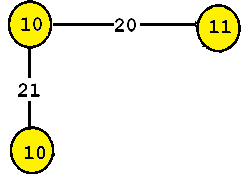This subgraph is frequent and is said to have a MNI support  (a frequency) of 2 since it appears twice in the input graphs according to the MNI support. The occurrences are:### Output file format

The output file format is defined as follows. It is a text file, listing all the frequent subgraphs found in the input graph database. A frequent subgraph is defined by a few lines of text that follow the following format:

• t # N * Z    This is the first line of a subgraph. It indicates that this is the N-th subgraph in the file and that its support is Z.
• v M L     This line defines the M-th vertex of the current subgraph, which has a label L
• e P Q L   This line defines an edge, which connects the P-th vertex with the Q-th vertex. This edge has the label L
• x X1 X2 ... This line lists the identifiers of all the graphs X1, X2 ... that contains the current subgraph.
For example, for the above example, the output is:

t # 0 * 2
v 0 10
v 1 11
v 2 10
e 0 1 20
e 0 2 21
x 0

If the optional parameter outputDebugInformation is activated (set to true) and outputGraphIds is activated as well, then additional information is included in the output. In that case the format is:

• t # N * Z *T    This is the first line of a subgraph. It indicates that this is the N-th subgraph in the file and that its support is Z. Moreover, it is indicates that the graph has T projections in the database)
• v M L     This line defines the M-th vertex of the current subgraph, which has a label L
• e P Q L   This line defines an edge, which connects the P-th vertex with the Q-th vertex. This edge has the label L
• x A1xB1 A2xB2 ... This line contains "x" followed by a list of pairs of the form AxB where A is the ID of a graph containing the subgraph, and B is the number of projections of the subgraph in graph A.

For example, for the above example the output when outputDebugInformation = true  and outputGraphIds = true, is :

t # 0 * 2 * 3
v 0 10
v 1 11
v 2 10
e 0 1 20
e 0 2 21
x 0x3

And if outputDebugInformation = true  and outputGraphIds = false, the output is :

t # 0 * 2 * 3
v 0 10
v 1 11
v 2 10
e 0 1 20
e 0 2 21

### Performance

The cgSpan algorithm is an important algorithm that is efficient and has inspired several other algorithms. This implementation is optimized and includes a few optimizations to increase efficiency.